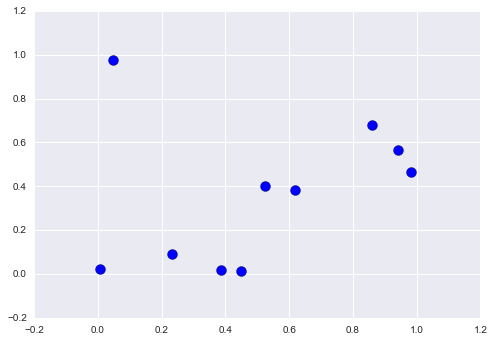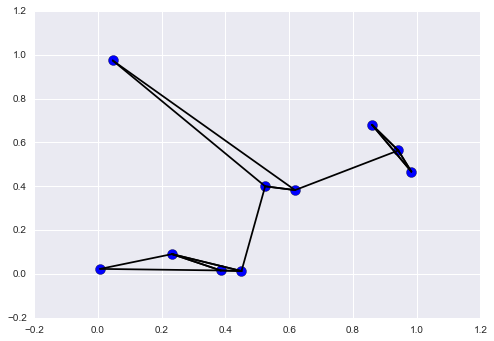## 9.10 数组排序

import numpy as np

def selection_sort(x):
for i in range(len(x)):
swap = i + np.argmin(x[i:])
(x[i], x[swap]) = (x[swap], x[i])
return x

x = np.array([2, 1, 4, 3, 5])
selection_sort(x)

# array([1, 2, 3, 4, 5])


def bogosort(x):
while np.any(x[:-1] > x[1:]):
np.random.shuffle(x)
return x

x = np.array([2, 1, 4, 3, 5])
bogosort(x)

# array([1, 2, 3, 4, 5])


### NumPy 中的快速排序：np.sort和np.argsort

x = np.array([2, 1, 4, 3, 5])
np.sort(x)

# array([1, 2, 3, 4, 5])


x.sort()
print(x)

# [1 2 3 4 5]


x = np.array([2, 1, 4, 3, 5])
i = np.argsort(x)
print(i)

# [1 0 3 2 4]


x[i]

# array([1, 2, 3, 4, 5])


#### 沿行或列的排序

NumPy 排序算法的一个有用特性是，能够使用axis参数来排序多维数组的特定行或列。例如：

rand = np.random.RandomState(42)
X = rand.randint(0, 10, (4, 6))
print(X)

'''
[[6 3 7 4 6 9]
[2 6 7 4 3 7]
[7 2 5 4 1 7]
[5 1 4 0 9 5]]
'''

# 排序 X 的每一列
np.sort(X, axis=0)

'''
array([[2, 1, 4, 0, 1, 5],
[5, 2, 5, 4, 3, 7],
[6, 3, 7, 4, 6, 7],
[7, 6, 7, 4, 9, 9]])
'''

# 排序 X 的每一行
np.sort(X, axis=1)

'''
array([[3, 4, 6, 6, 7, 9],
[2, 3, 4, 6, 7, 7],
[1, 2, 4, 5, 7, 7],
[0, 1, 4, 5, 5, 9]])
'''


### 部分排序：分区

x = np.array([7, 2, 3, 1, 6, 5, 4])
np.partition(x, 3)

# array([2, 1, 3, 4, 6, 5, 7])


np.partition(X, 2, axis=1)

'''
array([[3, 4, 6, 7, 6, 9],
[2, 3, 4, 7, 6, 7],
[1, 2, 4, 5, 7, 7],
[0, 1, 4, 5, 9, 5]])
'''


### 示例：K 最近邻

X = rand.rand(10, 2)


%matplotlib inline
import matplotlib.pyplot as plt
import seaborn; seaborn.set() # 绘图风格
plt.scatter(X[:, 0], X[:, 1], s=100);dist_sq = np.sum((X[:, np.newaxis, :] - X[np.newaxis, :, :]) ** 2, axis=-1)


# 对于每一对点
# 计算坐标的差
differences = X[:, np.newaxis, :] - X[np.newaxis, :, :]
differences.shape

# (10, 10, 2)

# 计算坐标的差
sq_differences = differences ** 2
sq_differences.shape

# (10, 10, 2)

# 对坐标差求和来获取距离平方
dist_sq = sq_differences.sum(-1)
dist_sq.shape

# (10, 10)


dist_sq.diagonal()

# array([ 0.,  0.,  0.,  0.,  0.,  0.,  0.,  0.,  0.,  0.])


nearest = np.argsort(dist_sq, axis=1)
print(nearest)

'''
[[0 3 9 7 1 4 2 5 6 8]
[1 4 7 9 3 6 8 5 0 2]
[2 1 4 6 3 0 8 9 7 5]
[3 9 7 0 1 4 5 8 6 2]
[4 1 8 5 6 7 9 3 0 2]
[5 8 6 4 1 7 9 3 2 0]
[6 8 5 4 1 7 9 3 2 0]
[7 9 3 1 4 0 5 8 6 2]
[8 5 6 4 1 7 9 3 2 0]
[9 7 3 0 1 4 5 8 6 2]]
'''


K = 2
nearest_partition = np.argpartition(dist_sq, K + 1, axis=1)


plt.scatter(X[:, 0], X[:, 1], s=100)

# 绘制每个点到它的两个最近邻的直线
K = 2

for i in range(X.shape):
for j in nearest_partition[i, :K+1]:
# 绘制 X[i] 到 X[j] 的直线
# 使用一些 zip 魔法来实现
plt.plot(*zip(X[j], X[i]), color='black')